## Example Questions

### Example Question #1 : Sequences

The first term in a sequence of integers is 2 and the second term is 10. All subsequent terms are the arithmetic mean of all of the preceding terms. What is the 39th term?

600

5

6

300

1200

6

Explanation:

The first term and second term average out to 6. So the third term is 6. Now add 6 to the preceding two terms and divide by 3 to get the average of the first three terms, which is the value of the 4th term. This, too, is 6 (18/3)—all terms after the 2nd are 6, including the 39th. Thus, the answer is 6.

### Example Question #5 : Sequences

Consider the following sequence of integers:

5, 11, 23, 47

What is the 6th element in this sequence?

95

191

93

189

191

Explanation:

First, consider the change in each element.  Notice that in each case, a given element is twice the preceding one plus one:

11 = 2 * 5 + 1

23 = 11 * 2 + 1

47 = 23 * 2 + 1

To find the 6th element, continue following this:

The 5th: 47 * 2 + 1 = 95

The 6th: 95 * 2 + 1 = 191

### Example Question #2 : How To Find The Nth Term Of An Arithmetic Sequence

The sequence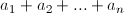begins with the numbers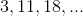and has the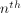term defined as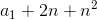, for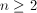.

What is the value of the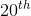term of the sequence?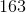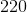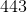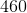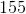Explanation:

The first term of the sequence is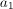, so here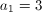, and we're interested in finding the 20th term, so we'll use n = 20.

Plugging these values into the given expression for the nth term gives us our answer.and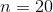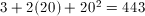### Example Question #7 : Sequences

In a sequence of numbers, the first two values are 1 and 2. Each successive integer is calculated by adding the previous two and mutliplying that result by 3. What is fifth value in this sequence?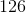Explanation:

Our sequence begins as 1, 2.

Element 3: (Element 1 + Element 2) * 3 = (1 + 2) * 3 = 3 * 3 = 9

Element 4: (Element 2 + Element 3) * 3 = (2 + 9) * 3 = 11 * 3 = 33

Element 5: (Element 3 + Element 4) * 3 = (9 + 33) * 3 = 42 * 3 = 126

### Example Question #8 : Sequences

Let Z represent a sequence of numbers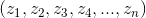wherein each term is defined as seven less than three times the preceding term.  If, what is the first term in the sequence?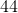Explanation:

Let us first write the value of a consecutive term in a numerical format: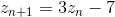Consequently,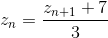Using the first equation, we can define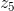in terms of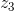: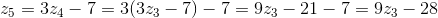This allows us to rewrite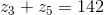as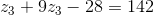Rearrangement of terms allows us to solve for: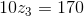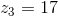Now, using our second equation, we can find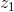, the first term: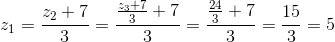Tired of practice problems?

Try live online GRE prep today.